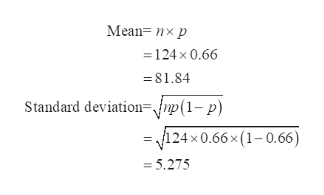# find the mean, variance, and standard deviation of the binomial distribution with the given values of n and pn=124, p=0.66

Question
2 views

find the mean, variance, and standard deviation of the binomial distribution with the given values of n and p

n=124, p=0.66

check_circle

Step 1

A binomial distribution can be used when the outcomes of certain variables can be divided into two categories: success and failure. The outcomes should be independent of each other.

The mean of a binomial distribution is:

Step 2

The standard deviation of a binomial distribution is,

Step 3

Here, n denotes the fixed number of trails, p denotes the probability of success.

Given:  n = 124, p=...help_outlineImage TranscriptioncloseMean= nx p 124 x 0.66 =81.84 Standard deviation{np(1- p) =24 x0.66x (1-0.66) =5.275 fullscreen

### Want to see the full answer?

See Solution

#### Want to see this answer and more?

Solutions are written by subject experts who are available 24/7. Questions are typically answered within 1 hour.*

See Solution
*Response times may vary by subject and question.
Tagged in

### Other